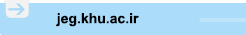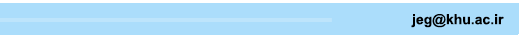[Home ] [Archive]   [ فارسی ]Main About Current Issue Archive Search Submit ContactVolume 14, Issue 1 (5-2020)2020, 14(1): 175-202 Back to browse issues page
Application of 3D numerical simulation to process the plate loading test results-Beheshtabad dam as a case study
Saeed Mahdavi *1, Mehrnosh Haghighat, Maryam Mokhtari
1- , smahdevari@cc.iut.ac.ir
Abstract:   (1451 Views)
Introduction
Material and Methods
The dam site is placed approximately at a distance of 2.7 km from the intersection of Koohrange and Beheshtabad river. In accordance with geological studies, the rocks in the site could be categorized in four units combined of Dolomite, Dolomitic Limestone, Limestone, Marl and Marly Limestone. Applying empirical equation the rock mass modulus of dam abutments is evaluated based on the laboratory test results and rock mass engineering classification systems. In addition, ASTM D4394 is applied to investigate the results of ten plate loading tests which are executed in the right and left abutments. To interpret the plate loading test results in the right abutment, a three-dimensional Fast Lagrange Analysis of Continuum (FLAC3D) model is developed.
Result and Discussion
To process the numerical simulation results, back analysis as a data processing tool is used. In this approach, the input parameters of numerical model will be changed in the way that the measured quantities by extensometers at the monitoring points are almost equal with the computed ones via numerical model at the corresponding points. Based on the sensitivity analysis carried out on the Mohr-Coulomb failure criterion parameters, the friction coefficient and cohesion variation do not affect the displacements calculated via numerical simulation as the more portion of gallery displacements are elastic. The error function is minimum when the rock mass modulus is 12 GPa and the horizontal to vertical stress ratio (K0) is equal to 0.5. The evaluated rock mass modulus based on the numerical simulation is two times lower than corresponding one evaluated applying empirical equation as a result of empirical equation uncertainty. Consideration of stress decrement under loading plate shows lower level of stress decrement under loading plate in ASTM D4394 compared to numerical simulation. This is why, the rock mass modulus, calculated based on ASTM D4394, increases dramatically by getting distance from the loading plate.
Conclusion
The empirical methods estimating the modulus of deformation based on rock mass classification systems tend to evaluate large value of modulus especially for the weak massive rocks.
As a result of galleries dimensions and semi-infinite boundary condition assumed in ASTM D4394, the calculated rock mass modulus increases dramatically by getting distance from loading plate. Therefore, the numerical simulation was applied to process the plate loading test results. A new normalized error function was developed based on measured displacements and the rock mass modulus in the right abutment was determined 12 GPa which is very lower than the calculated value using ASTM D 4394. Also, as a result of numerical simulation, the rock mass is uniform. The stress increment perpendicular to the loading plate was calculated applying numerical simulation which is 0-90 percent lower than those suggested by ASTM D 4394.

Type of Study: Original Manuscript | Subject: Geotecnic
Received: 2018/08/15 | Accepted: 2019/02/2 | Published: 2020/06/9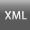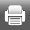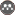Rights and permissionsThis work is licensed under a Creative Commons Attribution-NonCommercial 4.0 International License.